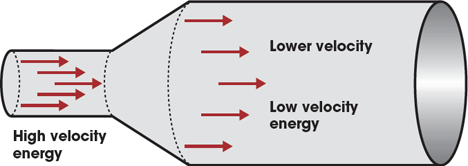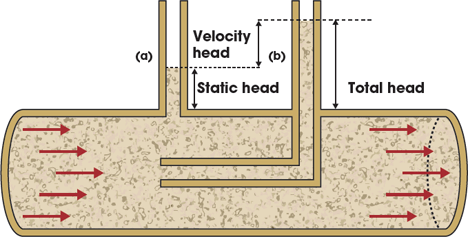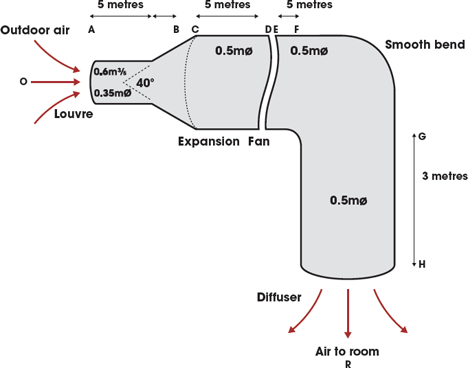# Module 31: Airflow pressure drop in HVAC ductwork

Fans consume a great deal of energy, and so their efficiency is crucial to preventing waste. The airflow through ducted systems is key to maintaining maximum efficiency. This CPD takes a detailed look at flow and pressure requirements for such systems.

It is somewhat odd that despite the universal adoption of fans in industrial, commercial (and increasingly domestic) systems, the underlying concepts that determine the size, selection and efficiency are still uncertain to many. It is thought that fans consume more than 20% of the electricity in buildings, and so are excellent candidates for optimisation when seeking opportunities to reduce the carbon footprint and the operating cost in the built environment.

This CPD will consider the flow and pressure requirements to allow air flow through ducted systems. A future CPD will apply this to consider the pressure profile through the whole system and the appropriate selection of fans.

### The ‘total’ story

When examining the air flow through a duct, it is convenient to consider the pressures in the flowing air in terms of duct static, velocity and total pressure. The development of these concepts comes from a standard relationship, the Bernoulli Equation, which applies the conservation of energy to incompressible flowing fluids. The equation (for a ‘frictionless’ system) is:

Potential Energy + Pressure Energy + Kinetic Energy = Constant (or Total Energy)

The potential energy relates to the elevation of the fluid (for example, its height above a datum such as ground floor level – think of the energy required to carry a barrel of water up several flights of stairs); pressure energy is due to the force of the fluid all around it (air at the bottom of a cold liftshaftwill have a pressure energy related to the force imposed by the weight of the column of air above it); and kinetic energy relates to the movement of the fluid (to the square of the fluid velocity).

As the sum of the three is constant in a closed system such as a duct or pipe (ignoring friction and assuming incompressible fluids) it means that if one of the values changes, then one or both of the others must compensate to keep the sum of the three constant.

So, for example if a round duct very gradually expands (as in Figure 1) the velocity goes down as the area of the duct increases. The potential energy stays the same (as the centreline of the duct is still at the same elevation) and hence the pressure energy must increase to compensate, for the loss in velocity energy.Figure 1: Air moving through gradually expanding duct

Air is, of course, compressible but, at the pressures experienced in HVAC ductwork, it is assumed that the air will not compress and, if the temperature does not vary, the density of the air (kg/cu m) will remain constant as it flows throughout the duct. This reasonable assumption also allows the use of water (in this article) to more readily illustrate the pressures involved in fluid flow. Drawing (a) in Figure 2 shows a round pipe carrying water with a section of clear tube attached at right angles (‘normally’) to the side of the pipe.Figure 2: Measuring the (a) static head and (b) total head of water flowing through a pipe

Looking at drawing (a) with the water flowing smoothly through the straight pipe, the height, z, of the column of fluid gives what is known as the ‘static head’ of the water at that point – the vertical pipe is a simple ‘manometer’. This reflects the static energy in the water, since the movement of the water is in line with the direction of the pipe and so the velocity of the water will not impose a pressure at the entry to this manometer tube.

If, as an example, the height of the water in the manometer, z, was 0.20m, then this would be the value of the static head and the static pressure (relative to the air around the pipe) at that point can be determined from pressure = ρ g z (Pa), where ρ is the density of the water, (nominally 1000kg/m3) and g is the acceleration due to gravity, 9.81 m/s2.

So, in this particular case the (relative) static pressure = ρ g z = 1000 x 9.81 x 0.20 = 1962Pa.

And these can readily be converted into static and velocity pressures, as before, using pressure = ρ g z. The manometer cannot reflect the ‘potential pressure’ – in a level piece of pipework, potential pressure will not alter but as the pipe rises the potential pressure will also rise and there will be an equivalent drop in the static pressure.

For the flow of air in ductwork in low-rise buildings the changes in potential pressure are almost always neglected, as these are relatively small (due to the low density of the air). So practically, when considering the pressure of ducted air systems, the potential pressure is assumed to be constant; duct velocity pressure (pv) + duct static pressure (ps) = duct total pressure (pt).

If the average velocity, c, of the air (m/s) is known, the value of velocity pressure (pv) can be calculated from 0.5 ρ c2 (and frequently this is shortened to 0.6 c2 by applying a ‘standard’ density of air of 1.2 kg/ m3). This value is based on air flow, with the speed c being measured normally to the direction of the air’s travel (ie in line with the duct) – in most ‘real’ applications the velocity of the air will alter across a duct due to the friction at the side of the duct, to obstructions and changes in direction. Pressure (pv) + duct static pressure (ps) = duct total pressure (pt), where the term ‘duct static pressure’ will be taken to mean ‘duct static pressure relative to the surrounding air’.

If the average velocity, c, of the air (m/s) is known, the value of velocity pressure (pv) can be calculated from 0.5 ρ c2 (frequently this is shortened to 0.6 c2 by applying a ‘standard’ density of air of 1.2 kg/m3).

In a theoretical world, if the duct was frictionless, then at two points in a duct (for example, points A and B in Figure 3) Bernoulli’s Equation would mean that the total pressure at A would equal the total pressure at B, ie, ptA = ptB. But, of course in real ducts there is friction and so ptA = (ptB + frictional losses).Figure 3: An example of a simplified ducted air system√

### Resistance to change

As the air enters the ductwork system – in Figure 3 through a simple louvred entry – the air will be accelerated from the still air outside the louvre to a velocity determined by the volume flowrate, q v (m3/s) of the air, and the area, A (m2) of the duct. In this case as c = q v /A = 0.6/0.1 = 6m/s. Before the air enters the duct it has a velocity pressure, p v , of virtually zero and once in the duct p v = 0.6×62 = 21.60Pa. This gain in velocity pressure will need to be matched by a drop in static pressure.

As the air flows through the louvre it will have to overcome some resistance and hence suffer an additional pressure loss. This can be calculated using the zeta factor, ζ, for the fitting (obtainable from section 4.11 of CIBSE Guide C 2007 or from manufacturers’ data).

The resulting drop in static pressure is given by Δps = ζ x pv, and the value of pv is normally taken as that downstream of a fitting (but this may vary and should be clearly indicated in the tables in Guide C and elsewhere). The ζ for this particular louvre (taken from the table in Guide C) is 3.0, so the static pressure loss as the air flows through the louvre due to friction, Δps = 3.0 x 21.60 = 64.80Pa.

Although expressed as a drop in static pressure, this loss will be a direct reduction in total pressure and, increasingly, many people relate the pressure drop in ducted air systems directly in terms of total pressure. This is useful for clarity when selecting fans, but the use of the static pressure can provide a clearer interpretation of the pressure inside the duct that is available to drive air out through supply terminals (such as diffusers and VAV boxes).

And so combining the louvre pressure loss with that required to match the gain in velocity pressure, the static pressure at a point just after point A would be -21.60- 64.80 = -85.40Pa

### Straight but still resistant

As the air flows through a straight duct (between points B and C) the friction of the air against the side walls of the duct (as well as between the air molecules themselves) will cause the air to lose energy (to heat and a little in producing sound).

The drop in energy manifests itself as a pressure drop. Between B and C the air will be travelling at constant velocity, so its velocity pressure will remain constant. The drop in pressure will be quite small (in a low velocity ductwork system) – typically around 1 Pa per metre run of straight ductwork. In this particular circular steel ductwork system the value is 1.3Pa/m (and this may be checked by using figure 4.3 in Guide C). The length of that duct is 5m, and so the pressure drop is 5 x 1.3 = 6.50Pa.

### Static regain

The next section is an expansion – in a real application the duct may change shape to pass around an obstacle, to join a device (such as a fan) or, indeed, the expansion may be there to alter the pressure characteristics in the duct.

Again, looking at Guide C (Table 4.55) the ζ factor for this expansion can be determined as ζ = 0.20 (where AreaC/AreaB = 0.20/0.10 = 3 and the angle of the ‘cone’ is 40°). In this case (for the expansion) the pressure loss is calculated using the entering air velocity pressure, ie 21.60Pa. So the pressure loss is 0.20 x 21.60 = 4.32Pa.

However, an interesting change takes place at the same time. As the air passes through the expansion, the velocity will drop to 0.6/0.2 = 3.00m/s giving p v = 0.6×3.002 =5.40Pa. Hence the velocity pressure has dropped by 21.60-5.40 = 16.20Pa. The loss in velocity pressure will be balanced by a gain in static pressure. So remembering that the pressure drop due to friction/turbulence will be 4.32Pa, the overall change in static pressure will be -4.32 + 16.20 = +11.88Pa, ie, an increase in static pressure – this is known as ‘static regain’.

For the time being the fan will be ignored (to be considered in a future article) so between C and F will be the straight 0.50 m diameter duct of 10 metres length. Again looking at duct sizing diagrams (in Guide C or elsewhere) this gives a pressure drop of 0.21Pa/m duct. So the pressure drop for this straight section is 10 x 0.21 = 2.10Pa.

### Final bend and away

The following bend is treated in just the same way as with any other duct fitting. Determine the value of ζ from tables and, if there is a change in duct area, determine the change in velocity pressure.

To determine the correct data in Guide C needs the Reynolds number, Re, for the air flow in the fitting. This is fairly straightforward as Re = ρ c d / η where d is the diameter of the duct (m) and η is the dynamic viscosity of the air (kg/ms). At around 20C the dynamic viscosity of air is approximately 18×10-6 kg/ms. So in this case, with the air velocity remaining at 3m/s, Re = 1.2 x 3 x 0.5/(18 x 10-6) = 1 x 105.

Using this value the ζ value can be read from the Guide C tables for a smooth 90° bend as ζ = 0.290 and hence the pressure loss calculated as 0.290 x 5.4Pa = 1.57Pa, and since there is no change in average velocity the velocity pressure remains at 5.40Pa. The pressure drop for the following straight section (G to H) is simply 3 x 0.21 = 0.63Pa (as per previous straight section).

Finally the air is supplied into the room. If this were just a plain opening at the end of the duct the static pressure at this point immediately before the air leaves the duct would be practically zero (ie, the same as the room or atmospheric pressure) and the duct total pressure would simply be due to the duct velocity pressure. This would effectively mean that there would be one velocity pressure loss as the air left the duct and passed into the room.

However, there would more normally be a diffuser or grille at the end of the duct (and frequently some flexible duct and/ or a reducer) that will additionally incur a pressure drop related to its ζ factor (or a pressure drop taken from manufacturers’ data). It is important not to forget the final velocity pressure, as it can be significant (although in this case it would only be 5.4Pa).

In a CPD article in the near future, this knowledge will be further applied to consider the system pressure profiles and fan requirements.